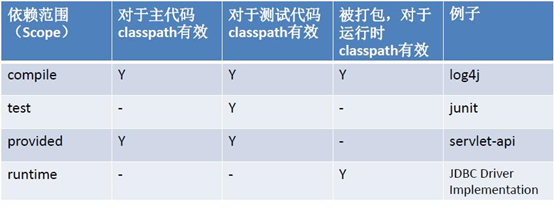# Monthly Archives: May 2021

First of all, we can have below schema for the players and its score:

contest_id(partitionKey), user_id1(sortKey), problem1_finish_time, problem2_finish_time, score(secondaryIndex)

For the score and user_id, we can build a BST. Let’s say we want to find the players who ranks between 50-60. We can find the 50th player in O(logN) time. Check this leetcode problem. Then, we can do extra 10 range scan based on the searched element.

Implementation can be done with redis ZADD, ZRANGE

Below is the command we

```0.0.0.0:6379> zadd players 400 d
(integer) 1
0.0.0.0:6379> zadd players 500 e
(integer) 1```

Here is a full list of the result with scores:

```0.0.0.0:6379> zrange players 0 -1 withscores
1) “b”
2) “100”
3) “a”
4) “200”
5) “c”
6) “300”
7) “d”
8) “400”
9) “e”
10) “500”```

By running ZRANGE board_name from to, it returns the result:

```0.0.0.0:6379> zrange players 2 4
1) "c"
2) "d"
3) "e"```

Check this post https://redislabs.com/solutions/use-cases/leaderboards/

## Maven scope: compile, test, provided, runtime## How to calculate percentile metrics

Suppose we want to calculate latency in p90 percentile.

1. Use count min sketch to count each latency times. For example, 5ms happened 10 times, 9ms happened 20 times etc2. Because latency has long tail. That means most of latency is around 7ms, but there could be cases for 100ms, 110ms etc. For that long tail latency, we kinda ignore don’t care the difference between 100ms and 101ms. So when the latency goes long, we bucketize it. Such as:Below is based on assumption that each metrics has 2000 tags combination. Each metrics has[max, min, count, sum, avg, p90, p95, p99, p99.9] dimensions.

# Calculate 1min sketch bucket storage

Assume each bucket range has 2% difference.

Calculate 1ms to 1min, how many bucket do we need? 1ms, 2ms, …, 1000. 1000 intervals
aq, aq^2, aq^3, …, aq^n.
1*(1.02)^n = 1000. Then we have n = 250

Calculate 1nano to 1day, how many bucket do we need? 1 day = 86,400s, 1s = 10^9s. So 1 day =10^9 * 10^5 = 10^14
(1.02)^n = 10^14.
Then we have n = 350 buckets

Each counter is 8 bytes. For 1min calculating, space it requires is 8*350=3KB.

Considering there are 2000 tags combination, it requires 2000*3KB = 6MB.

# Calculating Aggregated metrics storage

After 1 min is done, we can release the 6MB, and allocate a new one for next 1 min calculation.

For each min, after aggregating the results, we have [max, min, count, sum, avg, p90, p95, p99, p99.9]. 2000 tags combination. Each counter takes 8 bytes. So storage for 1 min needed is 9*2000*8bytes
=144KB

For 3 months, it takes 90*3600*144KB = 18GB.

## Aws cli, put/get kinesis record

```AWS_ACCESS_KEY_ID=xxxx AWS_SECRET_ACCESS_KEY=xxxx AWS_SESSION_TOKEN=xxxx \
aws kinesis put-record \
--region us-east-1 \
--stream-name \${streamName} \
--data "{\"pengliid\": \"pengli\",\"value\": [\"1\", \"2\", \"3\", \"4\"]}" \
--partition-key pengli-id```

In the output, remember the sequence number and shardId

```AWS_ACCESS_KEY_ID=xxxx AWS_SECRET_ACCESS_KEY=xxxx AWS_SESSION_TOKEN=xxxx \
aws kinesis get-shard-iterator \
--region us-east-1 \
--stream-name \${streamName} \
--shard-id \${shard_id} \
--shard-iterator-type AT_SEQUENCE_NUMBER \
--starting-sequence-number \${sequence_number}```

remember the shardIterator

```AWS_ACCESS_KEY_ID=xxxx AWS_SECRET_ACCESS_KEY=xxxx AWS_SESSION_TOKEN=xxxx \
aws kinesis get-records \
--region us-east-1 \
--shard-iterator \${shardIterator}```

Output is base64 format. Transform it.# Kinesis get-records shard-iterator

```command1: aws kinesis get-records --shard-iterator xxxxxx
return:
{
records: [record1]
nextiterator: yyyyyyy
}

command2: aws kinesis get-records --shard-iterator yyyyyyy
return:
{
records: [record2]
nextiterator: zzzzzzz
}```
Category: aws

## Cache TTL consideration

1. When there is no eviction mechanism, we can use ttl to invalidate. But this is based on that cache might have stale data.
2. TTL doesn’t lead 100% cache correctness. If we want to guarantee cache correctness, we need evection mechanism, write-through etc strategies.
3. In theory, TTL is just a way of data replacement. Others are LRU, LFU.

## MVCC

To solve non-repeatable read:
1. lock
2. mvcc

As long as there is an update, then generate a new record with current tx_id. undo log points to previous one.

When read, it should have:

```ReadView {
m_ids_list,
min_trx_id,
max_trx_id,
creator_trx_id
}```

When read or doing a transaction, it should have current tx_id and its ReadView. Go to the record, check if the top record’s tx_id is smaller than the tx_id in ReadView. If so, then this is the value for it. If not, check the next record in undo log…

Good article for MVCC.

https://blog.csdn.net/flying_hengfei/article/details/106965517

## hungarian algorithm template

```public int findMaxMatch(boolean[][] graph) {
int match = 0;
int[] match = new int[graph.length];

for (int i = 0; i < graph.length; i++) {
boolean[] visited = new int[graph.length];
if (find(i, graph, match, visited)) {
match++;
}
}
return match;
}

// match[j], for each record j in B set, it matches to a certain record in A set.
boolean find(int i, boolean[][] graph, int[] match, boolean[] visited) {
for (int j = 0; j < graph.length; j++) {
if (!graph[i][j] || visited[j]) {
continue;
}
visited[j] = true;
if (match[j] == -1 || find(match[j], graph, match, visited)) {
match[j] = i;
return true;
}
}
return false;
}```

## binary search templatehttps://youtu.be/JuDAqNyTG4g?t=802

practice lc785

## payment system keywowrd

double payment
sync payment
async payment
3rd party interaction
different state
sensitive data, accuracy, relational database.

websocket, long polling, stateful